# 向量数学¶

## 坐标系统(2D)¶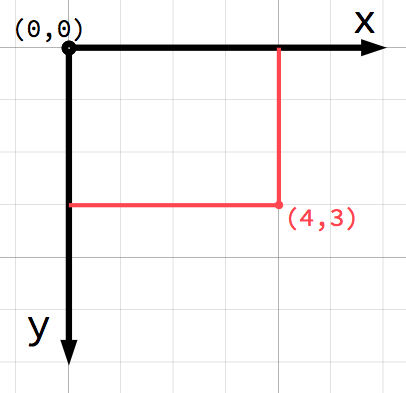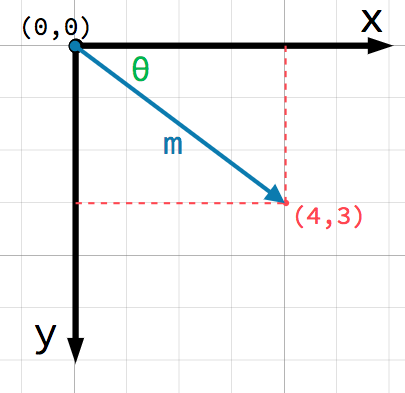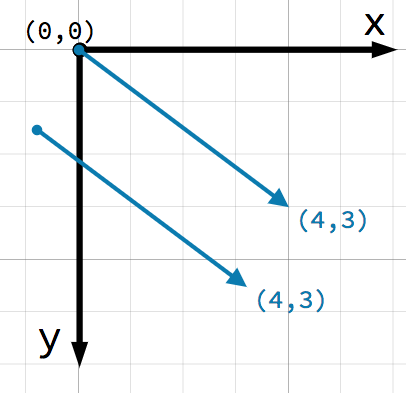## 向量运算¶

You can use either method (x and y coordinates or angle and magnitude) to refer to a vector, but for convenience, programmers typically use the coordinate notation. For example, in Godot, the origin is the top-left corner of the screen, so to place a 2D node named Node2D 400 pixels to the right and 300 pixels down, use the following code:

\$Node2D.position = Vector2(400, 300)

var node2D = (Node2D) GetNode("Node2D");
node2D.Position = new Vector2(400, 300);


Godot supports both Vector2 and Vector3 for 2D and 3D usage, respectively. The same mathematical rules discussed in this article apply to both types.

### 成员访问¶

# create a vector with coordinates (2, 5)
var a = Vector2(2, 5)
# create a vector and assign x and y manually
var b = Vector2()
b.x = 3
b.y = 1

// create a vector with coordinates (2, 5)
var a = new Vector2(2, 5);
// create a vector and assign x and y manually
var b = new Vector2();
b.x = 3;
b.y = 1;


### 添加向量¶

var c = a + b  # (2, 5) + (3, 1) = (5, 6)

var c = a + b;  // (2, 5) + (3, 1) = (5, 6)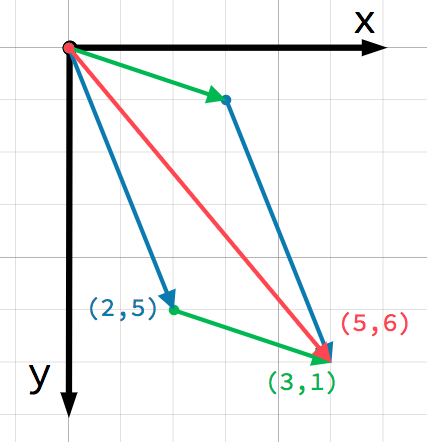### 标量乘法¶

var c = a * 2  # (2, 5) * 2 = (4, 10)
var d = b / 3  # (3, 6) / 3 = (1, 2)

var c = a * 2;  // (2, 5) * 2 = (4, 10)
var d = b / 3;  // (3, 6) / 3 = (1, 2)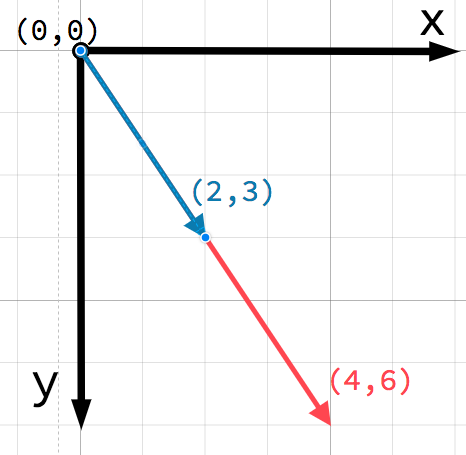## 实际应用¶

### 移动¶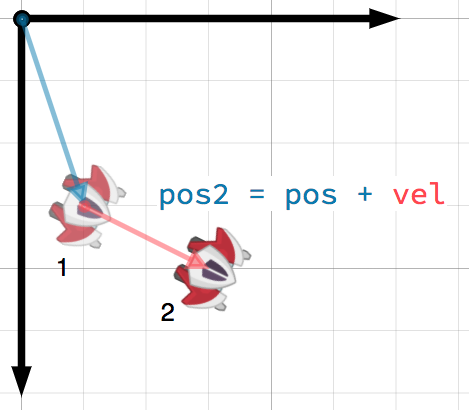### 指向一个目标¶## 单位向量¶

### 归一化¶

var a = Vector2(2, 4)
var m = sqrt(a.x*a.x + a.y*a.y)  # get magnitude "m" using the Pythagorean theorem
a.x /= m
a.y /= m

var a = new Vector2(2, 4);
var m = Mathf.Sqrt(a.x*a.x + a.y*a.y);  // get magnitude "m" using the Pythagorean theorem
a.x /= m;
a.y /= m;


a = a.normalized()

a = a.Normalized();


### 反射¶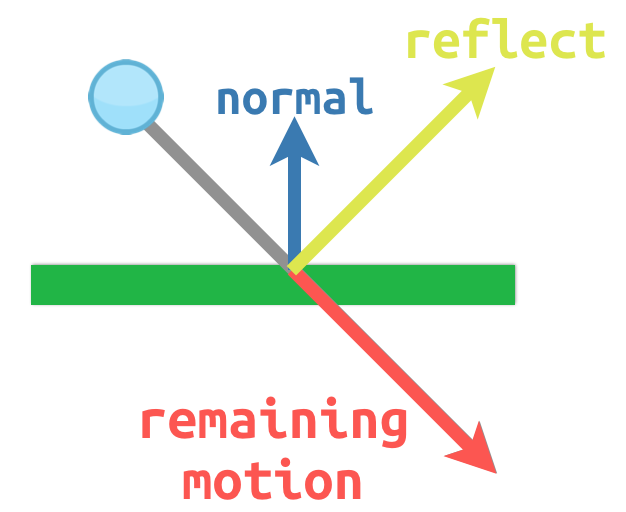# object "collision" contains information about the collision
var collision = move_and_collide(velocity * delta)
if collision:
var reflect = collision.remainder.bounce(collision.normal)
velocity = velocity.bounce(collision.normal)
move_and_collide(reflect)

// KinematicCollision2D contains information about the collision
KinematicCollision2D collision = MoveAndCollide(_velocity * delta);
if (collision != null)
{
var reflect = collision.Remainder.Bounce(collision.Normal);
_velocity = _velocity.Bounce(collision.Normal);
MoveAndCollide(reflect);
}


## 点乘¶var c = a.dot(b)
var d = b.dot(a)  # these are equivalent

float c = a.Dot(b);
float d = b.Dot(a);  // these are equivalent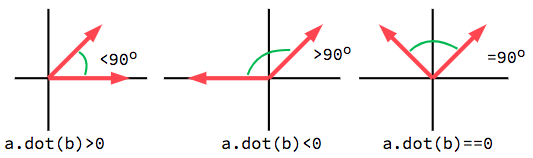### 面向问题¶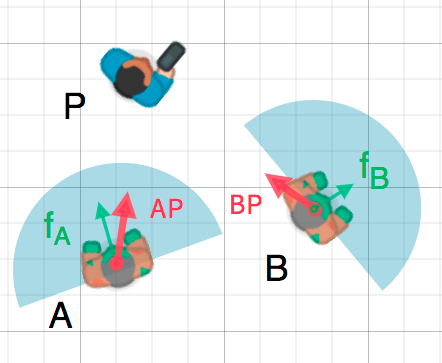var AP = (P - A).normalized()
if AP.dot(fA) > 0:
print("A sees P!")

var AP = (P - A).Normalized();
if (AP.Dot(fA) > 0)
{
GD.Print("A sees P!");
}


## 叉乘¶

Like the dot product, the cross product is an operation on two vectors. However, the result of the cross product is a vector with a direction that is perpendicular to both. Its magnitude depends on their relative angle. If two vectors are parallel, the result of their cross product will be a null vector.var c = Vector3()
c.x = (a.y * b.z) - (a.z * b.y)
c.y = (a.z * b.x) - (a.x * b.z)
c.z = (a.x * b.y) - (a.y * b.x)

var c = new Vector3();
c.x = (a.y * b.z) - (a.z * b.y);
c.y = (a.z * b.x) - (a.x * b.z);
c.z = (a.x * b.y) - (a.y * b.x);


var c = a.cross(b)

var c = a.Cross(b);


### 法线计算¶

func get_triangle_normal(a, b, c):
# find the surface normal given 3 vertices
var side1 = b - a
var side2 = c - a
var normal = side1.cross(side2)
return normal

Vector3 GetTriangleNormal(Vector3 a, Vector3 b, Vector3 c)
{
// find the surface normal given 3 vertices
var side1 = b - a;
var side2 = c - a;
var normal = side1.Cross(side2);
return normal;
}


### 指向目标¶

In the dot product section above, we saw how it could be used to find the angle between two vectors. However, in 3D, this is not enough information. We also need to know what axis to rotate around. We can find that by calculating the cross product of the current facing direction and the target direction. The resulting perpendicular vector is the axis of rotation.